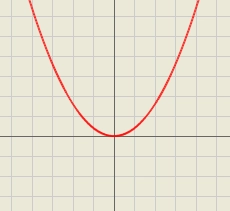# Determine 80662

Given the function y = x2 - 4x + 3. Determine all real numbers z such that g(x) = g(-2).

x1 =  6
x2 =  -2

### Step-by-step explanation:Did you find an error or inaccuracy? Feel free to write us. Thank you!

Tips for related online calculators
Are you looking for help with calculating roots of a quadratic equation?
Do you have a linear equation or system of equations and looking for its solution? Or do you have a quadratic equation?

#### Grade of the word problem:

We encourage you to watch this tutorial video on this math problem: